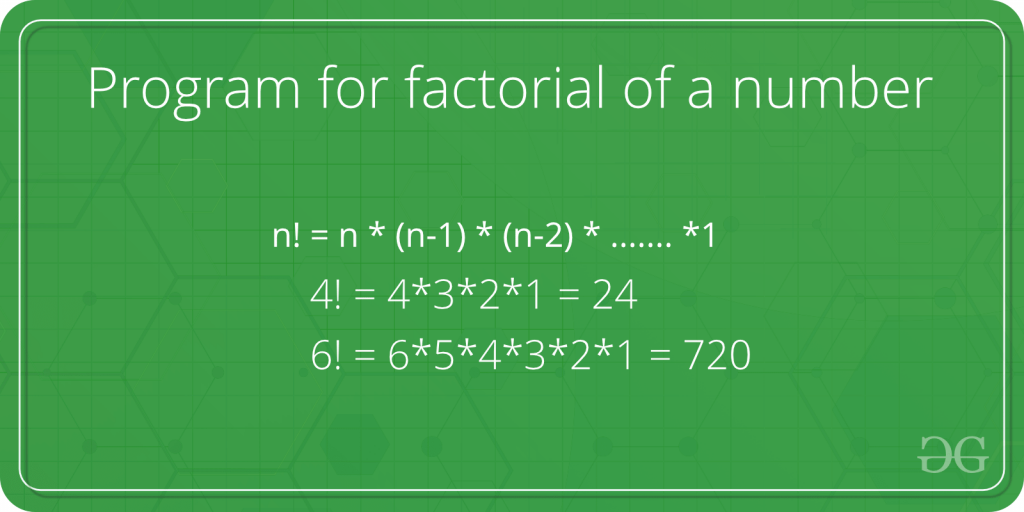# Factorial of 100### Factorial of hundred:

The factorial of a positive integer n is the product of all the positive integers from 1 to n. For example, the factorial of 4 is 4! = 4 * 3 * 2 * 1 = 24. The factorial of 100 is 100! = 100 * 99 * 98 * … * 2 * 1. This is a very large number, equal to approximately 9.3 x 10^157. It has 158 digits! Here is the first few digits of 100!:

eg: $4! = 4 \times 3 \times 2\times 1 = 24$

###### 100!

$100! = 100 \times 99 \times 98 \times 97 \times …….. 4 \times 3 \times 2\times 1$ = $93326215443944152681699238856266700490715968264381621468592963895217599993229915$ $608941463976156518286253697920827223758251185210916864000000000000000000000000$

The factorial of a positive integer n is the product of all the positive integers from 1 to n. For example, the factorial of 4 is 4! = 4 * 3 * 2 * 1 = 24. The factorial of 100 is 100! = 100 * 99 * 98 * … * 2 * 1. This is a very large number, equal to approximately 9.3 x 10^157. It has 158 digits! Here is the first few digits of 100!:

### Factorial of 0! = 1 Proof

The factorial of 0, written as 0!, is defined to be equal to 1. This may seem counterintuitive at first, but there are a few different ways to justify this definition.

One way to think about it is to consider the factorial function as a way of counting the number of ways to arrange a certain number of objects. For example, if you have 3 objects, there are 3! = 6 ways to arrange them: (1, 2, 3), (1, 3, 2), (2, 1, 3), (2, 3, 1), (3, 1, 2), and (3, 2, 1). If you have no objects, there is only 1 way to arrange them: the empty set, or “nothing.”

Another way to justify the definition of 0! as 1 is to consider the definition of the factorial function as a product of all the positive integers from 1 to n. When n = 0, there are no positive integers to include in the product, so the product is equal to 1.

It is also possible to define 0! as the limiting value of n! as n approaches 0. This can be shown using mathematical techniques, such as L’Hopital’s rule. However, this definition relies on advanced mathematical concepts and may not be as intuitive as the other explanations.